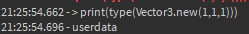# Variable in a coroutine can't change out of scope?

While creating a simple cooldown function with coroutines, It seems that I can’t change the debounce variable inside the actual function, I’m not sure if this is an intended behaviour or if I’m doing something wrong, either way, I would greatly appreciate any help.

``````--FUNCTION
local Cooldown = coroutine.wrap(function(Debounce, DebounceTimer)
wait(DebounceTimer)
Debounce = false
end)
``````
``````--I call the function like this
Cooldown(DodgeDebounce, DodgeDebounceTimer)
``````

I should also note that while trying to debug this, I printed out the debounce in the function scope and it printed false like I was hoping for, however when I print the debounce out of the scope, it prints true.

2 Likes

you can use a modulescript to do that or just create the function before then add it. such as:

``````local Debounce = true
function cooldown(Debounce, DebounceTimer)
wait(DebounceTimer)
print('epic')
Debounce = false
end

coroutine.wrap(function()
cooldown(true, 2)
end)()
``````
1 Like

Unfortunately I’m still having the same issues with the second method, as for the first one, the current script the function is on is already acting as my InputController, therefore the cooldown function most likely won’t be used anywhere else other than that script.

1 Like

Due to how I’ve constructed the framework for my game, I wouldn’t have a place to put the BoolValue object and even then, it wouldn’t match the way my game is structured and would honestly do me more harm than not.

1 Like

Then use a table so they pass by reference and not by value like this:

``````local DodgeDebounce = {true}
local Cooldown = coroutine.wrap(function(Debounce,DebounceTimer)
wait(DebounceTimer)
Debounce = false
end)
Cooldown(DodgeDebounce,5)
``````
2 Likes

Sorry, I don’t really understand. Can you elaborate?

1 Like

Fundamental datatypes are passed by value and not by reference in Lua. If you/the callee changes those parameters in the function it won’t change the variable passed by the caller. You either have to make the cooldown variable an upvalue or pass it in a datatype which is passed by reference, for example a table.

2 Likes

Oh I see, thanks for the useful information. Guess I learnt something new today.

1 Like

Basically value types like string, number or bool are pased by value which means when you do something like this:

``````local test = "Test1"
local test2 = test
test = "Test3"
print(test)
print(test2)

--Output
- Test3
- Test1
``````

You will notice that test2 variable copied the test variable so when the test variable changes test2 stayed the same.

However functions, tables and userdata are passed by reference and not value which means they don’t get copied unlike strings and numbers so when you do this:

``````local test = {"Test1"}
local test2 = test
test = "Test3"
print(test)
print(test2)
--Output
- Test3 (x2)
``````

You will see that they both output the same string because tables are passed by reference so when you do `local test2 = test` test2 just exists as a reference and references to test table when you use it.

3 Likes

Hmm it didn’t seem to work, I’ll look through everything a couple times just in case I missed something

1 Like

Interesting, just to clarify, would CFrames, Vector3’s and things like that all classify as userdata?

1 Like

They do count as userdata, you can check it yourself with a simple script:
`print(type(Vector3.new(1,1,1)))`2 Likes

Sounds good, thanks for the extra info.

1 Like

Whether values are passed byval or byref is mostly irrelevant. You can’t just change the value of a variable local to one scope and affect the value outside the scope as you could in say C++. As such, you can sort of imagine everything is passed byval but some types are mutable while others aren’t.

So when you pass a table to another function, you can edit that table’s fields, but not the variable which references the table itself.

``````local foo = { x = 5 }
local bar = foo

print(foo.x == bar.x) --> true

bar.x = 10
print(foo.x == bar.x) --> true

bar = { x = 20 }
print(foo.x == bar.x) --> false
``````

There’s two approaches you can take to solve this. One involves using the table for the state while the other uses the parent scope.

Using a state table:

``````local state = {
debounce = true
}

local cooldown = coroutine.wrap(function (state, debounceTimer)
wait(debounceTimer)
state.debounce = false
end
``````

Using the parents scope:

``````do -- create a new scope
local debounce = false
local cooldown = coroutine.wrap(debounceTimer)
wait(debounceTimer)
debounce = false -- note `debounce` isn't local to the coroutines scope
end
-- change the value of debounce here will change it inside of the coroutine
end
``````
2 Likes